# Trace Of Matrix

Matrix has a special function called trace function. If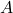is a square matrix then the sum of its main diagonal entry is called trace of matrixand is denoted by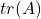.

Letbe a square matrix with size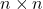, then

\begin{aligned}
A = \begin{bmatrix}a_{11} & a_{12} & a_{13}\\a_{21} & a_{22} & a_{23}\\a_{31} & a_{32} & a_{33}\end{bmatrix}
\end{aligned}

The trace of matrix is,

\begin{aligned}
tr(A) = a_{11} + b_{22} + c_{33}
\end{aligned}

Let is see few examples of traces of matrices.

Example #1

Let A be a square matrix of size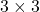\begin{aligned}
A = \begin{bmatrix}-1 & 4 & 2\\6 & 2 & 7\\5 & 1 & 8\end{bmatrix}
\end{aligned}

The trace of the matrix A is,

\begin{aligned}
&tr(A) = (-1) + 2 + 8 = 9\\\\
&tr(A) = 9
\end{aligned}

Example #2

Let B be a square matrix of size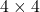.

\begin{aligned}
B = \begin{bmatrix}6 & 1 & 1 & -2\\5 & 9 & -1 & 3\\0 & 1 & 7 & 2\\3 & 7 & 8 & 5\end{bmatrix}
\end{aligned}

The trace of matrix B is,

\begin{aligned}
&tr(B) = 6  + 9 + 7 + 5 = 27\\\\
&tr(B) = 27
\end{aligned}

If the matrix A is not a square matrix, thenis not defined.